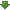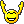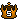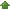TwinWolves
Would you like to react to this message? Create an account in a few clicks or log in to continue.

# CALLING ALL MATH GEEKS QQ (Algebra II here)##CALLING ALL MATH GEEKS QQ (Algebra II here)

[You must be registered and logged in to see this image.]

Jaehyo

Posts : 64
Join date : 2012-07-24

##Re: CALLING ALL MATH GEEKS QQ (Algebra II here)

Jaehyo wrote:[You must be registered and logged in to see this image.]

3^3 * y^4 * y^2=

27 * y^(4+2)=

27*y^6

Eisenmeteor

Posts : 27
Join date : 2012-07-14
Age : 28

##Re: CALLING ALL MATH GEEKS QQ (Algebra II here)

[You must be registered and logged in to see this image.]

Jaehyo

Posts : 64
Join date : 2012-07-24

##Re: CALLING ALL MATH GEEKS QQ (Algebra II here)

Jaehyo wrote:[You must be registered and logged in to see this image.]

3^4 x^2
81x^2

Kaze

Posts : 10
Join date : 2012-07-18

##Re: CALLING ALL MATH GEEKS QQ (Algebra II here)

Jaehyo wrote:[You must be registered and logged in to see this image.]

3^4 x^2
81x^2

Kaze

Posts : 10
Join date : 2012-07-18

##Re: CALLING ALL MATH GEEKS QQ (Algebra II here)

Jaehyo wrote:[You must be registered and logged in to see this image.]

(3^7 / 3^3) * (x^5 / x^3)

[3^(7-3)] * [x^(5-3)]

3^4 * x^2

81*x²

Eisenmeteor

Posts : 27
Join date : 2012-07-14
Age : 28

##Re: CALLING ALL MATH GEEKS QQ (Algebra II here)

[You must be registered and logged in to see this image.]

Jaehyo

Posts : 64
Join date : 2012-07-24

##Re: CALLING ALL MATH GEEKS QQ (Algebra II here)

2/3 a^7 b^1

6 (a^(3*3) b^(1*3))
3^2 a^(1*2) b^(1*2)

6 a^9 b^3
9 a^2 b^2

6/9 a^(9-2) b^(3-2)

2/3 a^7 b

Last edited by Kaze on Wed Aug 22, 2012 2:56 am; edited 1 time in total

Kaze

Posts : 10
Join date : 2012-07-18

##Re: CALLING ALL MATH GEEKS QQ (Algebra II here)

Jaehyo wrote:[You must be registered and logged in to see this image.]

6[a^(3*3)*b³] / (3²*a²*b²)

6(a^9*b³) / (9*a²*b²)

6/9 * (a^9) / a² * b³/b²

2/3 * a^7 * b

(2*a^7*b)/3

Eisenmeteor

Posts : 27
Join date : 2012-07-14
Age : 28

##Re: CALLING ALL MATH GEEKS QQ (Algebra II here)

[You must be registered and logged in to see this image.]

Jaehyo

Posts : 64
Join date : 2012-07-24

##Re: CALLING ALL MATH GEEKS QQ (Algebra II here)

Jaehyo wrote:[You must be registered and logged in to see this image.]

-1* (2² * x^4*2)/ (5² * y²)

-(4*x^8 )/(25y²)

Eisenmeteor

Posts : 27
Join date : 2012-07-14
Age : 28

##Booo~

2[3(b-5)-(b^2+b+3)]
what i did was distribute the three and negative sign, 2(3b-15-b^2-b-3)
now distribute the two, (6b-30-2b^2-2b-6)
add all the common numbers together, (4b-36-2b^2)
rearrange the numbers in order and answer is: -2b^2+4b-36
DONEif you have to simplify it more then take out the common factor of negative 2 to get : -2(b^2+2b-18)

Yaichi

Posts : 4
Join date : 2012-08-21
Age : 27

##Re: CALLING ALL MATH GEEKS QQ (Algebra II here)

[You must be registered and logged in to see this image.]
find an expression for the area of the shaded portion of the figure[b]

Jaehyo

Posts : 64
Join date : 2012-07-24

##Re: CALLING ALL MATH GEEKS QQ (Algebra II here)

Jaehyo wrote:[You must be registered and logged in to see this image.]
find an expression for the area of the shaded portion of the figure[b]

Shaded portion area = Whole area - White area

S = [3x(3x+10)]-[x*(x+4)]

S = (9x²+30x)-(x²+4x)

S = 8x²+26x

Eisenmeteor

Posts : 27
Join date : 2012-07-14
Age : 28

##How many jelly coins?

[You must be registered and logged in to see this link.]airmanof1
AlphaPosts : 69
Join date : 2012-07-14
Age : 42
Location : North Carolina USA

##slowpoke.jpg

erm... sry for necroing, either way deal with it. First and foremost for everyone having issues or simply looking for solutions for math problems visit [You must be registered and logged in to see this link.] and input whateve the math formula is and voila, computer magic is made. This is a hugely powerful tool that can solve diferencial equations among many other complex math formulas, just oughta put it out there. Also air... the music you used in that video...is perfect...

input example: ((3^7)*(x^5))/((3^3)(x^3)) this is for the second one you posted Jae. Do try it out, you have graphs, derivatives and whatever else you can think off done for you, and the free version even allows you to see the answer step by step ( they limit it to 3 times a day though ). Either way, it's a must if you have any advanced or simple math classes, so be sure to give it a try.Posts : 76
Join date : 2012-07-13
Age : 27
Location : Lamego

##Re: CALLING ALL MATH GEEKS QQ (Algebra II here)

ShadowDx wrote: erm... sry for necroing, either way deal with it. First and foremost for everyone having issues or simply looking for solutions for math problems visit [You must be registered and logged in to see this link.] and input whateve the math formula is and voila, computer magic is made. This is a hugely powerful tool that can solve diferencial equations among many other complex math formulas, just oughta put it out there. Also air... the music you used in that video...is perfect...

input example: ((3^7)*(x^5))/((3^3)(x^3)) this is for the second one you posted Jae. Do try it out, you have graphs, derivatives and whatever else you can think off done for you, and the free version even allows you to see the answer step by step ( they limit it to 3 times a day though ). Either way, it's a must if you have any advanced or simple math classes, so be sure to give it a try.

Thanks!

Jaehyo

Posts : 64
Join date : 2012-07-24

##Re: CALLING ALL MATH GEEKS QQ (Algebra II here)